One thing that is used by a large number of chemistry researchers is NMR (Nuclear Magnetic Resonance). In particular, laboratories that specialize in organic chemistry or need to determine the structure of compounds frequently use NMR.

There are two types of such NMR, 1H-NMR and 13C-NMR. 1H-NMR is the most commonly used NMR technique, and 13C-NMR is rarely used. Unless you’re studying compounds with complex structures, you won’t use 13C-NMR. In other words, you should only learn 1H-NMR at first.

So, what is the principle behind 1H-NMR? Even though nuclear magnetic resonance is used to study the structural formula of a compound, NMR only shows lines, and you need to read and understand the data.

So we will explain how to understand 1H-NMR.

## What Does It Mean to Create Energy with the Zeeman Effect?

In order to understand the principles of 1H-NMR, you need to learn about fields. This is because, in order to make a difference in energy, a field must exist.

For example, suppose you are moving into space. In this case, no matter what position you are in, there is no difference in energy. This is because, in space, there is no gravity. On earth, gravity is a field.

On earth, where there is a field, which has more energy, standing up or standing on one’s head? Naturally, the energy of standing on one’s head is higher than that of the other.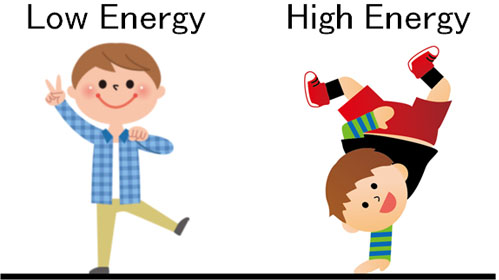On the other hand, what about the moon instead of the earth? Compared to the earth, the gravity of the moon is one-sixth. This means that the field energy is lower on the moon. Thus, if the field force is different, the energy possessed by matter will also be different.

Electrons have an electric charge, and electrons are rotating. When a charged material rotates, it emits a magnetic force as a result. A magnetic field is then applied. If a magnetic field is applied, the electron, a small magnet, will be in only two states.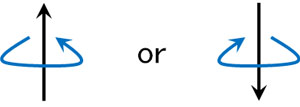The electron, which is a small magnet, will have an N-pole and an S-pole. As a result, when there is a magnetic field, the energy is high when the N and N poles are close together, and low when they are opposite.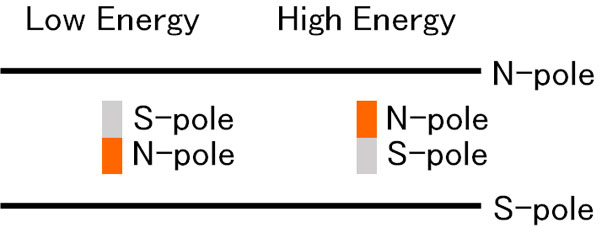In a strong magnetic field, the atomic spectrum changes. The resulting ability to read a number of spectra information is called the Zeeman effect.

Applying a magnetic field produces the Zeeman effect. As a result, electrons that were pointing in different directions are split into only two directions. Naturally, this is more often the case with smaller energy.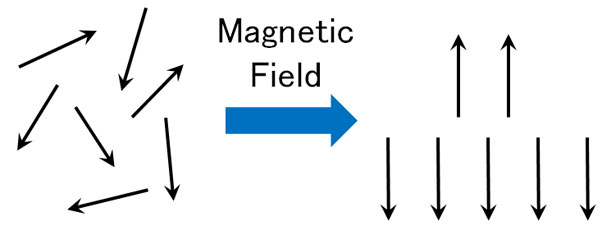However, there is a point where if you give energy externally while the Zeeman effect is happening, it all becomes high energy.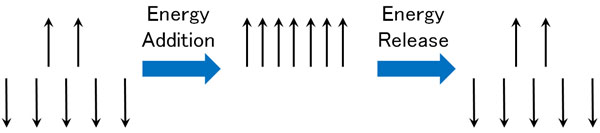After gaining energy, the electrons immediately release energy and return to their original state. We can then measure the point at which the 1H proton becomes energetic. This is how the spectrum appears as NMR data.

### Different Locations of Low and High Magnetic Fields Depending on the Electron Density (Shielding Effect)

When measuring the 1H proton, the properties of all hydrogen atoms are the same. Why do the spectra appear differently? This is because the electron density of each hydrogen atom is different. Understand that NMR (Nuclear Magnetic Resonance) is a tool that measures the differences in the electron density of hydrogen atoms.

For example, suppose that if you add energy of 10 to a hydrogen atom, it goes into a high-energy state for a fraction of a moment, and you can observe its spectrum. If the electron barrier is 3, you can observe the spectrum by giving it 13 energy.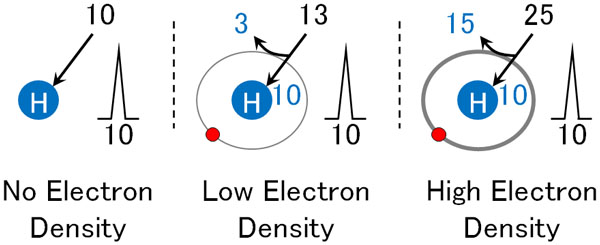In the same way, if the electron barrier is 15, you can observe the spectrum if you give it an energy of 25. The fact that the electron barrier blocks energy is called the shielding effect.

Hydrogen is bonded to atoms such as carbon and nitrogen; Since each proton (hydrogen atom) has a different electron density, the shielding effect changes where the peaks are observed.

For example, the structural formula of propanol is as follows.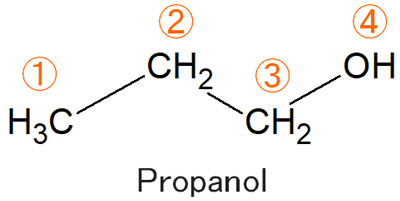With the second and third protons, which one would be observed at a lower magnetic field (lower energy)?

In the case of the second hydrogen atom, there are two carbon atoms next to it, which means that the electrons are being pushed, and the electron density is high.

On the other hand, the third proton has an oxygen atom next to it. The oxygen atom has a strong electronegativity, and the electrons are pulled toward the oxygen atom. As a result, the electron density is low. From these facts, you can expect the third proton to appear in a low magnetic field.

Naturally, the fourth proton, which is directly bound to the oxygen atom, will be observed in a lower magnetic field.

Simply put, you can think of NMR as a method to investigate the electron density around a hydrogen atom (proton). By checking the spectra, you can infer the structural formula of the compound.

For low and high magnetic fields, for example, the following NMR spectra are displayed.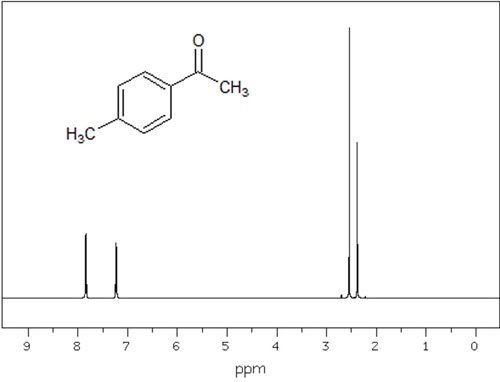In this way, we can see where the peaks are in low and high magnetic fields. Different compounds have different electron densities for each proton, which means that the peaks appear in different places.

## Important Coupling and Integral Values in NMR Signals

However, you can’t deduce the structural formula of a compound just by looking at where the spectra are located in the low and high magnetic fields. There are other things to consider in NMR: coupling and integration.

In addition to where the peaks appear, the shape and area of the peaks are also important. By examining these, you will be able to infer the structure of the compound.

We’ll explain how to think about each of these.

### Peak-Splitting Couplings (Non-Equivalent): Singlets and Doublets

When using an NMR (Nuclear Magnetic Resonance) instrument, hydrogen is usually observed as a single signal. For example, only one peak appears in the following conditions.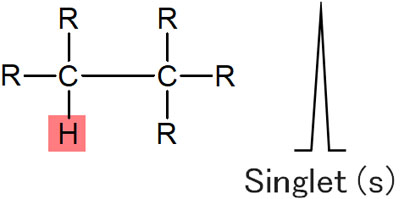This is called a singlet.

However, in NMR, it is affected by the hydrogen bond to the neighboring carbon. This is called coupling. For example, in the following compound, focus on the red hydrogen atom. In this case, the peak is a double line because there is a single hydrogen atom next to it.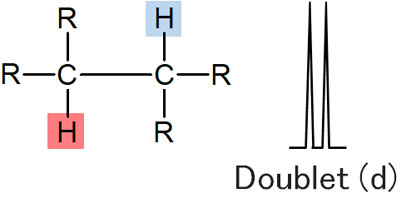A double line in NMR is called a doublet.

Then, what about a situation where there are two hydrogen atoms next to? In this case, the peak is further split into two, forming a quadruple line.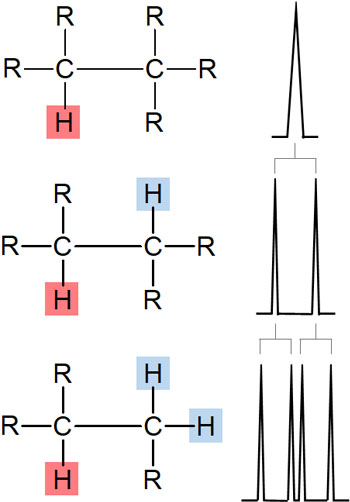Understand that if there is a hydrogen atom next to it, the peak will be split by the effect of the hydrogen atom.

### If the J-Values Are Equivalent: Triplet, Quartet, Quintet

However, normal compounds do not have peaks as described above. Earlier, I described a special state of non-equivalence. Instead, it is more common to think of equivalent states.

When a proton peak is split by 1H-NMR, the distance between the peaks is called the J-value (coupling constant). Unless there is a special reason, the J-value is the same when coupling occurs. This is called the equivalent state.

Now let’s consider the same situation as before, two hydrogen atoms next to it. Since the J-values are the same, the middle peak will overlap when coupling occurs. In the figure below, we assume that the J-value is 12 Hz.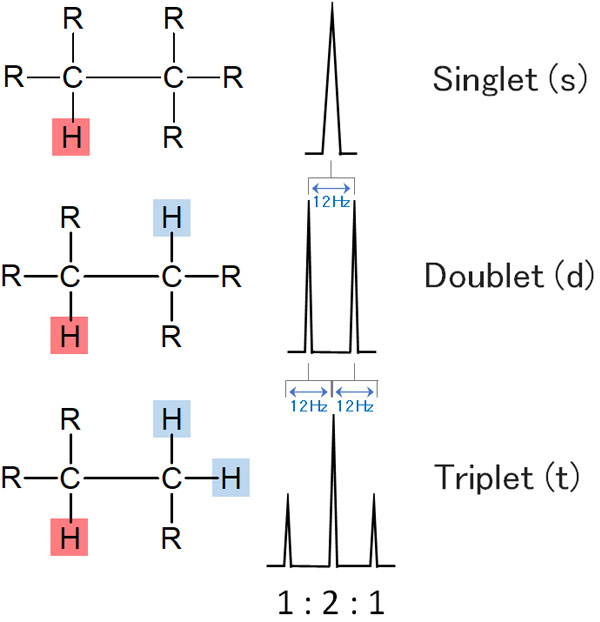The middle peak overlaps, resulting in a triple line of 1:2:1. This is called a triplet.

In the same way, with three hydrogen atoms next to it, you get a quadruple line. This is a quartet. And if there are four hydrogen atoms next to it, there will be a five-fold line. In NMR, this is called a quintet.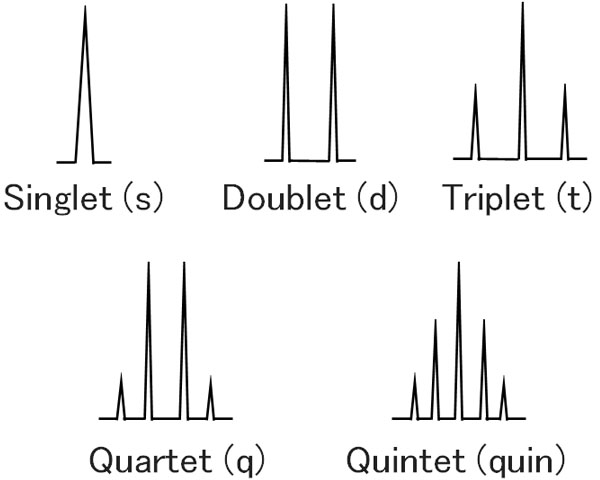In NMR, the peak splits due to the influence of the neighboring hydrogen.

-The Reason for Coupling

Why is it affected by the neighboring hydrogen in NMR? This is because the neighboring hydrogen has two spin states. Specifically, it can be in two states, ↑ and ↓. As a result, if there is one hydrogen next to it, it becomes a doublet.

On the other hand, if there are two hydrogen atoms next to it, what happens? In this case, there are four types of electron spin states.

• ↑ ↑
• ↑ ↓
• ↓ ↑
• ↓ ↓

However, ↑ ↓ and ↓ ↑ have the same energy. In other words, they can be considered the same. The result is a triplet with a 1:2:1 area ratio. This is why we have triplets, quartets and quintets.

### Compare the Integral Value (Integral Ratio) and Estimate the Number of Hydrogen

Furthermore, in NMR, you have to look at the integral value (integral ratio). Think of the integral value as the area of the NMR spectrum when it is observed. Depending on the area size, you can estimate the number of protons bonded to the carbon.

For example, suppose the following case. If you focus on the red hydrogen atom, the first case has one hydrogen. On the other hand, the second has two hydrogen atoms.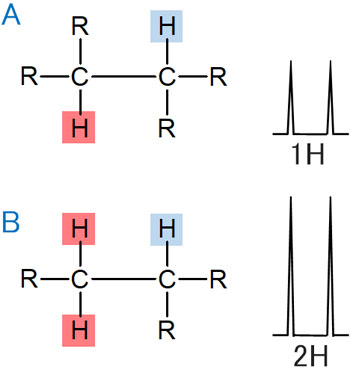The neighboring carbon has one hydrogen atom bonded, which can be observed in a doublet.

In this case, compared to A, B has twice the area. The reason for this is that A has one hydrogen atom, while B has two hydrogen atoms. Naturally, B has a larger integral value by that amount. From this, you can easily infer the number of protons bonded to the carbon.

When you measure it, you calculate the area ratio for each hydrogen as the integral value. By getting the integral value for each peak from the NMR spectrum and checking the area ratio (integral ratio), you can determine the structure.

## Solvents: Deuterium DMSO or Deuterium Chloroform

So what is the procedure when you use NMR to make a measurement? In this regard, the compound you want to measure must be dissolved in a solvent. The compound can never be measured as a solid, and because it’s a liquid, it can be split into two energies when a magnetic field is applied.

This means that you need a solvent to dissolve it. The most common solvents to dissolve are deuterium DMSO (dimethyl sulfoxide) and deuterium chloroform.

DMSO can dissolve most compounds. This makes it convenient, but DMSO is expensive. Therefore, if you are making multiple NMR measurements, your professor may frown at you if you use DMSO every time.

Chloroform, on the other hand, is cheap. Professors who don’t want to lose money will actively recommend the use of chloroform when making NMR measurements. However, in the case of chloroform, there are often compounds that are difficult to dissolve. In this case, there is no choice but to use DMSO.

In addition, the solvent is also a compound, and the solvent contains hydrogen. Therefore, you should understand that not only compounds you want to measure, but also DMSO and chloroform peaks will be observed. These are called solvent peaks. So you can ignore the solvent peaks in NMR.

-Deuterated Solvent (Deuterium), but Solvent Peaks Exist

Incidentally, the solvents used in deuterium DMSO and deuterium chloroform solvents are special liquids called a deuterated solvents. The deuterated solvent replaces ordinary hydrogen (1H) with deuterium (2H), which has a heavier mass than ordinary hydrogen.

Since this is measured by 1H-NMR, if the solvent that dissolves the compound remains 1H, only the solvent peak will be observed. This is because the amount of solvent is overwhelmingly greater than the amount of compound you want to measure. Therefore, by replacing the hydrogen with deuterium, the solvent peak is minimized to the extent possible. As a result, the compound peaks can be detected.

Of course, it is not possible to completely replace hydrogen with deuterium. So the solvent peaks will inevitably appear.

### TMS (Tetramethylsilane) Is the Reference Material for NMR: Zero Chemical Shift

Also, even though NMR is used to measure low and high magnetic fields, the reference point is not known. Therefore, it is necessary to clearly define where the zero point is. If you don’t compare it to something else, you won’t know if it’s a correct measurement.

That’s why TMS (tetramethylsilane) is always used as reference material in NMR. All you have to do is put a drop of this compound into the solution to be measured.

TMS (tetramethylsilane) has the following structure.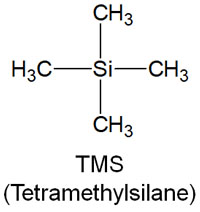TMS is a silicon compound and has methyl groups around the silicon atoms. As a result, the compound has a very high electron density. The point at which TMS is observed is considered to be zero in NMR, because the peak is only observed at fairly high energies.

The point where the TMS peak is observed is used as the reference (zero). This is why TMS is called a reference material. Compared to TMS, NMR observes where the peaks appear in low and high magnetic fields.

The position where the peaks appear is called the chemical shift; for TMS, the chemical shift is determined to be zero.

## NMR Is a Tool to Identify the Structural Formula of a Single Compound

When using NMR (Nuclear Magnetic Resonance), there is a major principle to follow. It is important to observe only a single compound, and not to measure a mixture of two or more compounds.

When two or more compounds are present, the NMR will show peaks of multiple compounds. As a result, it will not be possible to determine which compound the detected peaks belong to, and the structure cannot be determined.

Of course, in some cases, solvent peaks can be observed due to the presence of methanol or acetic acid in the solution. However, this is not a major problem because people who are familiar with research can quickly identify this problem.

It is easy to guess where the methanol and acetic acid peaks will appear and what form they will take.

However, NMR is used to measure unknown compounds. It is important to understand that if there are two or more unknown compounds with unknown structural formulas, it is impossible to determine their structures.

### Structures with Largely Complex Molecular Weights and Macromolecules such as Proteins are Difficult to Analyze

There are other points to keep in mind when using NMR to determine structures. Compounds with overly complex structures are more difficult to analyze.

NMR is a method for measuring the number of hydrogen atoms and the environment. Naturally, the more complex the structure is and the more protons (hydrogen atoms) there are, the more peaks to be observed. In addition, the number of observed peaks often overlaps. As a result, compounds with large molecular weights are more difficult to analyze.

When it comes to macromolecules such as proteins, structure determination is impossible. Even for compounds with large molecular weight, structure determination is difficult. For example, proteins have at least a molecular weight of 4,000.

1H-NMR is a tool for measuring the environment of hydrogen atoms. Therefore, it is an excellent tool for measuring low-molecular-weight compounds, and it is not suitable for determining the structure of compounds with large molecular weights. This is one of the disadvantages of using NMR for structure determination.

## Learn the Principles of NMR and Conduct Chemical Research

After a single compound has been extracted by synthesis or extraction, the next step is structure determination. To find out what kind of structure the target compound has, we use 1H-NMR to study the proton environment and determine the structure.

If you are in an organic chemistry lab, you will use NMR every day. We need to know if the compound we synthesized is the target compound or not.

Also, in the laboratory to extract unknown compounds, you use NMR to determine the structure. The structural formula is inferred from NMR measurement data.

In chemical research, NMR is used frequently. So you need to learn the principles of NMR. Let’s learn about it, including why we use NMR.